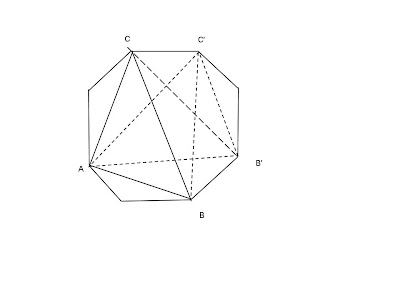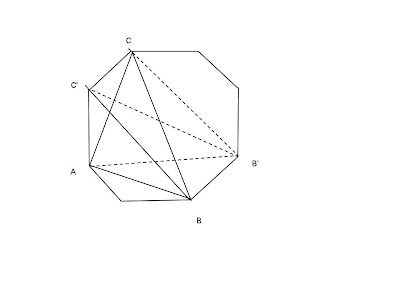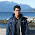## Thursday, August 18, 2011

### Maximum Triangle Area (Part 2)

I hope you enjoyed the part 1. In part - 1. We came up to an algorithm of complexity O(n ^ 2 log n). We'll try to improve it now.

Our problem is now reduced to: Given a convex polygon having n points. Find three points from these n points which form the largest triangle area.

Let's consider our points are ordered in counter clockwise and named P0, P1, ... , Pn - 1.

Let's start with A = P0, B = P1, C = P2. Now we will be moving C counter clockwise order (i.e. P3, P4, ...), as long as the triangle area doesn't decrease. And we know(from part 1), the area will not increase anymore after this point. So let's stop moving C. Let's say, we stopped C at Pk. So we found the largest triangle, having A = P0, and B = P1. Now it's time to move B. Let's move B to the adjacent point clockwise, if the area doesn't decreases. Now for new AB we have to find the best position of C, so that area is maximized. We will find that, we don't need to iterate from the next point of B, rather we can proceed with C as where it is now and try to move it counter clockwise as long the area doesn't decrease. Will it work always? Let's consider that it will not work, i.e. There will be a case where for a given AB, we will find maximum triangle ABC, and after moving B to B', we will find maximum triangle AB'C', where C' comes before C (By C' comes before C, I mean if we count points of the polygon starting from A, C' will come before C), and AB'C' > ABC, as the following picture.In the image above, we got ABC as the largest triangle for base AB. And after moving B to B', we got the largest triangle AB'C', where C' comes before C.
we know that, ABC >= ABC' ...  (As ABC is the largest triangle for base AB)
Now,
ABC' + ACC' = ABC'C = ABC + BCC'
=> ACC' >= BCC'  (as ABC >= ABC' from )

From (2), we can say, ACC' >= B'CC' (We already told for a given base, AB, if we move another point C, it will increase area of ABC up to a certain point and than start decreasing, i.e. it will never increase again after that point. Here for base CC', it started decrease from point A to B, so it will not increase from B to B'. As ACC' >= BCC', so ACC' >= B'CC').

ACC' >= B'CC'
=> ACC' + AB'C' >= B'CC' + AB'C' (adding AB'C' in both side)
=> AB'C'C >= B'CC' + AB'C'
=> AB'C + B'CC' >= B'CC' + AB'C'
=> AB'C >= AB'C'

So, for base AB' any point C' before C will not increase the area. So we can safely iterate from current position of C to find a larger area triangle. After finding the best position of C, move B again, if it increases the area. If moving B doesn't increases the area, stop moving B. Question is, is there any chance of getting larger triangle don't stop moving B in this case? Let's consider that there is a chance to get a larger area.For example, in the above picture, for base AB we found ABC as the largest triangle, but after moving B to B' we found AB'C < ABC. Now for base AC, we already know that there area will not increase any more by moving B. And we also proved that area will not increase moving C in clockwise. So only way left is moving C counter clockwise. Let's say we found a solution AB'C', where C' comes after C. As this is a better solution, AB'C' > AB'C

Now, ABC > ABC' (If ABC' >= ABC, we would have already moved C to C' for base AB).
=> ABC + CC'A > ABC' + CC'A
=> ABCC' > ABC' + CC'A
=> ABC' + CC'B > ABC' + CC'A
=> CC'B > CC'A 

And, AB'C' > AB'C
=> AB'C' + CC'B' > AB'C + CC'B
=> AB'CC' > AB'C + CC'B
=> AB'C + CC'A > AB'C + CC'B
=> CC'A > CC'B 

 and  are contradictory. So if after moving B to B', the area decreases, it will not possible to get larger area for the same A. So we have to stop moving B, and this ABC is the largest triangle for a given A. As B can move up to n times and also C can move up to n times. So for a given A, our algorithm complexity is O(2n) ~ O(n). We have to do this for each possible position of A. So our algorithm becomes O(n ^ 2).

Hopefully you enjoyed it so far. We'll try to improve more in part 3.

1.Great analysis! I am loving it so much! Waiting for the part 3.

2.Thanks. I'll tr to post it soon.

3.A question regarding . Could you explain the first step (written below)?

> And, AB'C' > AB'C
> => AB'C' + CC'B' > AB'C + CC'B

4.Adding CC'B to both sides.

1.CC'B' was added to the left side of inequality and CC'B was added to the right one. B and B' are different points. Or am I missing somewhere a proof that these areas are equal?

5.After second part, our algorithm became something like this -
for each 0 <= i < n, initialize A = Pi, B = P(i + 1) % n, C = P(i + 2) % n, then move C counter clockwise until area non-decreasing, move B to its adjacent point counter clockwise, if area non-decreasing, move C again until area non decreasing and so on. You will get the maximum area for each A and output the maximum of these areas. For a given point starting point Pi, if the maximum area is ABC. We can say ABC is 'stable' triangle for A, in other word s(pi, Pi + 1, Pi + 2) = ABC, that means we cannot move B or C any further by not decreasing the area.

6.Here, dp[oldCount + 1][oldSum + cur] += dp[oldCount][oldSum] / (n - oldCount) * (oldCount + 1); because, after having a permutation of oldCount I can add a new person in this permutation in (oldCount + 1) possible positions. So we're multiplying (oldCount + 1), and probability of being selected at (oldCount + 1)th person from (n - oldCount) person is 1 / (n - oldCount). So we're multiplying this also. All f(count, sum) can be counted in O(n * n * p). As we're iterating over all the blockers. So the overall algorithm will be O(n ^3 * p).